# User:Tohline/Appendix/Ramblings/ConcentricEllipsodalCoordinates

(Difference between revisions)
 Revision as of 20:03, 27 October 2020 (view source)Tohline (Talk | contribs) (→Other Coordinate Pair in the Tangent Plane)← Older edit Revision as of 20:14, 27 October 2020 (view source)Tohline (Talk | contribs) (→Primary (radial-like) Coordinate)Newer edit → Line 75: Line 75: \hat\imath \biggl[ x(x^2 + q^2 y^2 + p^2 z^2)^{- 1 / 2} \biggr] \hat\imath \biggl[ x(x^2 + q^2 y^2 + p^2 z^2)^{- 1 / 2} \biggr] + \hat\jmath \biggl[ q^2y(x^2 + q^2 y^2 + p^2 z^2)^{- 1 / 2} \biggr] + \hat\jmath \biggl[ q^2y(x^2 + q^2 y^2 + p^2 z^2)^{- 1 / 2} \biggr] - + \hat\jmath \biggl[ p^2 z(x^2 + q^2 y^2 + p^2 z^2)^{- 1 / 2} \biggr] + + \hat{k}\biggl[ p^2 z(x^2 + q^2 y^2 + p^2 z^2)^{- 1 / 2} \biggr] [/itex] [/itex] Line 91: Line 91: \hat\imath \biggl( \frac{x}{\lambda_1} \biggr) \hat\imath \biggl( \frac{x}{\lambda_1} \biggr) + \hat\jmath \biggl( \frac{q^2y}{\lambda_1} \biggr) + \hat\jmath \biggl( \frac{q^2y}{\lambda_1} \biggr) - + \hat\jmath \biggl(\frac{p^2 z}{\lambda_1} \biggr) \, , + + \hat{k}\biggl(\frac{p^2 z}{\lambda_1} \biggr) \, , [/itex] [/itex]

# Concentric Ellipsoidal (T6) Coordinates

## Background

Building on our general introduction to Direction Cosines in the context of orthogonal curvilinear coordinate systems, and on our previous development of T3 (concentric oblate-spheroidal) and T5 (concentric elliptic) coordinate systems, here we explore the creation of a concentric ellipsoidal (T6) coordinate system. This is motivated by our desire to construct a fully analytically prescribable model of a nonuniform-density ellipsoidal configuration that is an analog to Riemann S-Type ellipsoids.

## Orthogonal Coordinates

We start by defining a "radial" coordinate whose values identify various concentric ellipsoidal shells,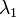$~\lambda_1$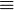$~\equiv$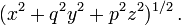$~(x^2 + q^2 y^2 + p^2 z^2)^{1 / 2} \, .$

When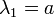$~\lambda_1 = a$, we obtain the standard definition of an ellipsoidal surface, it being understood that,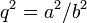$~q^2 = a^2/b^2$ and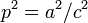$~p^2 = a^2/c^2$. (We will assume that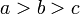$~a > b > c$, that is,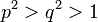$~p^2 > q^2 > 1$.)

A vector,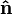$~\bold{\hat{n}}$, that is normal to the$~\lambda_1$ = constant surface is given by the gradient of the function,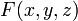$~F(x, y, z)$$~\equiv$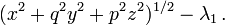$~(x^2 + q^2 y^2 + p^2 z^2)^{1 / 2} - \lambda_1 \, .$

In Cartesian coordinates, this means,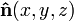$~\bold{\hat{n}}(x, y, z)$$~=$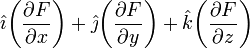$~ \hat\imath \biggl( \frac{\partial F}{\partial x} \biggr) + \hat\jmath \biggl( \frac{\partial F}{\partial y} \biggr) + \hat{k} \biggl( \frac{\partial F}{\partial z} \biggr)$$~=$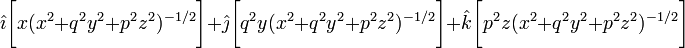$~ \hat\imath \biggl[ x(x^2 + q^2 y^2 + p^2 z^2)^{- 1 / 2} \biggr] + \hat\jmath \biggl[ q^2y(x^2 + q^2 y^2 + p^2 z^2)^{- 1 / 2} \biggr] + \hat{k}\biggl[ p^2 z(x^2 + q^2 y^2 + p^2 z^2)^{- 1 / 2} \biggr]$$~=$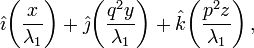$~ \hat\imath \biggl( \frac{x}{\lambda_1} \biggr) + \hat\jmath \biggl( \frac{q^2y}{\lambda_1} \biggr) + \hat{k}\biggl(\frac{p^2 z}{\lambda_1} \biggr) \, ,$

where it is understood that this expression is only to be evaluated at points,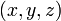$~(x, y, z)$, that lie on the selected$~\lambda_1$ surface — that is, at points for which the function,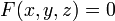$~F(x,y,z) = 0$. The length of this normal vector is given by the expression,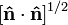$~[ \bold{\hat{n}} \cdot \bold{\hat{n}} ]^{1 / 2}$$~=$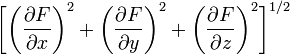$~ \biggl[ \biggl( \frac{\partial F}{\partial x} \biggr)^2 + \biggl( \frac{\partial F}{\partial y} \biggr)^2 + \biggl( \frac{\partial F}{\partial z} \biggr)^2 \biggr]^{1 / 2}$$~=$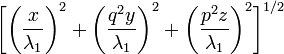$~ \biggl[ \biggl( \frac{x}{\lambda_1} \biggr)^2 + \biggl( \frac{q^2y}{\lambda_1} \biggr)^2 + \biggl(\frac{p^2 z}{\lambda_1} \biggr)^2 \biggr]^{1 / 2}$$~=$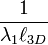$~ \frac{1}{\lambda_1 \ell_{3D}}$

where,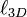$~\ell_{3D}$$~\equiv$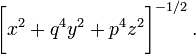$~\biggl[ x^2 + q^4y^2 + p^4 z^2 \biggr]^{- 1 / 2} \, .$

It is therefore clear that the properly normalized normal unit vector that should be associated with any$~\lambda_1$ = constant ellipsoidal surface is,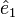$~\hat{e}_1$$~\equiv$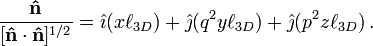$~ \frac{ \bold\hat{n} }{ [ \bold{\hat{n}} \cdot \bold{\hat{n}} ]^{1 / 2} } = \hat\imath (x \ell_{3D}) + \hat\jmath (q^2y \ell_{3D}) + \hat\jmath (p^2 z \ell_{3D}) \, .$

From our accompanying discussion of direction cosines, it is clear, as well, that the scale factor associated with the$~\lambda_1$ coordinate is,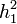$~h_1^2$$~=$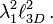$~\lambda_1^2 \ell_{3D}^2 \, .$

We can also fill in the top line of our direction-cosines table, namely,

 Direction Cosines for T6 Coordinates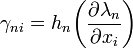$~\gamma_{ni} = h_n \biggl( \frac{\partial \lambda_n}{\partial x_i}\biggr)$$~n$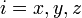$~i = x, y, z$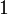$~1$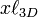$~x\ell_{3D}$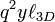$~q^2 y \ell_{3D}$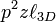$~p^2 z \ell_{3D}$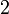$~2$ --- --- ---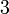$~3$ --- --- ---

### Other Coordinate Pair in the Tangent Plane

Let's focus on a particular point on the$~\lambda_1$ = constant surface,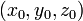$~(x_0, y_0, z_0)$, that necessarily satisfies the function,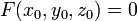$~F(x_0, y_0, z_0) = 0$. We have already derived the expression for the unit vector that is normal to the ellipsoidal surface at this point, namely,$~\hat{e}_1$$~\equiv$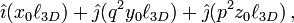$~ \hat\imath (x_0 \ell_{3D}) + \hat\jmath (q^2y_0 \ell_{3D}) + \hat\jmath (p^2 z_0 \ell_{3D}) \, ,$

where, for this specific point on the surface,$~\ell_{3D}$$~=$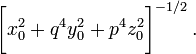$~\biggl[ x_0^2 + q^4y_0^2 + p^4 z_0^2 \biggr]^{- 1 / 2} \, .$

The two-dimensional plane that is tangent to the$~\lambda_1$ = constant surface at this point is given by the expression,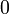$~0$$~=$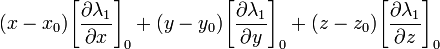$~ (x - x_0) \biggl[ \frac{\partial \lambda_1}{\partial x} \biggr]_0 + (y - y_0) \biggl[\frac{\partial \lambda_1}{\partial y} \biggr]_0 + (z - z_0) \biggl[\frac{\partial \lambda_1}{\partial z} \biggr]_0$$~=$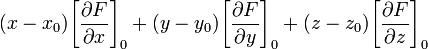$~ (x - x_0) \biggl[ \frac{\partial F}{\partial x} \biggr]_0 + (y - y_0) \biggl[\frac{\partial F}{\partial y} \biggr]_0 + (z - z_0) \biggl[ \frac{\partial F}{\partial z} \biggr]_0$$~=$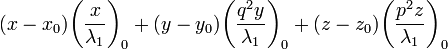$~ (x - x_0) \biggl( \frac{x}{\lambda_1}\biggr)_0 + (y - y_0)\biggl( \frac{q^2 y }{ \lambda_1 } \biggr)_0 + (z - z_0)\biggl( \frac{ p^2z }{ \lambda_1 } \biggr)_0$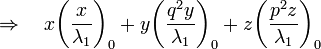$~\Rightarrow~~~ x \biggl( \frac{x}{\lambda_1}\biggr)_0 + y \biggl( \frac{q^2 y }{ \lambda_1 } \biggr)_0 + z \biggl( \frac{ p^2z }{ \lambda_1 } \biggr)_0$$~=$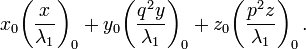$~ x_0 \biggl( \frac{x}{\lambda_1}\biggr)_0 + y_0 \biggl( \frac{q^2 y }{ \lambda_1 } \biggr)_0 + z_0 \biggl( \frac{ p^2z }{ \lambda_1 } \biggr)_0 \, .$